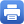Columbia Home
Number Theory

Number theory is one of the oldest branches of mathematics and is concerned with the properties of numbers in general. In the past few decades, research in number theory has progressed at a rapid rate on many fronts. Recently, important new results have arisen from analytic, geometric, and p-adic methods. These advances have been used to bring about breakthroughs, solve longstanding problems, and raise new inspiring questions.

Analytic number theory is the study of the distribution of prime numbers. One of the most important unsolved problems in mathematics is the Riemann hypothesis about the zeros of the Riemann zeta function, which gives a square root type error term for the number of primes in a large interval. An important extension of Riemann’s zeta function is the notion of automorphic L-functions which are Mellin transforms of automorphic forms. The theory of multiple Dirichlet series (Dirichlet series in several complex variables) introduced in 1980’s is now emerging as an important tool in obtaining sharp growth estimates for zeta and L-functions, an important classical problem in number theory with applications to algebraic geometry. One of the greatest applications of Grothendieck’s theory of schemes is Deligne’s proof of the Riemann hypothesis for L-functions for varieties over finite fields (which was first formulated by Weil). Thanks to the profound insight of Langlands, now embodied in the Langlands program: there is a sweeping vision of connections between automorphic L-functions on the one hand, and motivic L-functions, on the other. This vision encompasses the Artin and Shimura-Taniyama conjectures, both of which played a key role in Wiles’ proof of Fermat last theorem. The main technique of Wiles, the deformation of Galois representations, is a new direction, now quite extensively developed, which falls outside the scope of the Langlands program proper.

Arithmetic geometry is the study of integer or rational solutions of systems of polynomial equations using geometric methods. It turns out that the nature of the solution depends on the underlying complex varieties. For a curve of genus 0, the problem is completely local by the Hasse-Minkowski theorem, and can be solved by the quadratic reciprocity law. The Mordell-Weil Theorem and Faltings Theorem give the finiteness of rational points on curves of genus 1 and larger. The proof of these theorems uses a theory of heights i.e., Arakelov geometry. In this theory, Grothendieck’s theory of schemes is naturally combined with complex geometry to provide a very fine tool to study intersections of arithmetic cycles. A crucial question which remains open today is the effectivity of solutions. The ABC conjecture and its refinements provide some effective bounds for curves. For elliptic curves, one has the Birch and Swinnerton-Dyer (BSD) conjecture which relates the Mordell-Weil group and the central values of L-series arising from counting rational points over finite fields. The Gross-Zagier formula gives an expression for the heights of CM-points in terms of the central derivatives for L-functions. This formula together with an Euler system argument provides substantial evidence supporting the BSD conjecture.Print this page